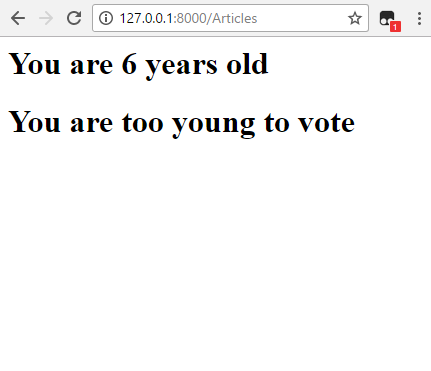﻿ How to Add an If Statement to a Template in Django ﻿# How to Add an If Statement to a Template in DjangoSo, templates are a major part of Django.

When you're building a website in Django, you almost undoubtedly use templates, many times, which are HTML files that usually include CSS files, to style the webpage, which is typical of any web page.

So, the template file will have HTML and CSS, normally.

However, many times, the web pages aren't static. They offer dynamic content.

So, many times, we want to add Python to template files like HTML pages, so that we can generate dynamic content.

One such thing we can do to template files is add if statements to a template file.

How we add if statements to a template in Django is shown below.

We are going to randomly generate a number from 1 to 100.

We're going to create an if, elif, else script so that if the number is below 18, we print out, "You are too young to vote." If the number is equal to 18, then we print out, "You have just reached the age to vote". If the number is greater than 18, we print out, "You are old enough to vote"

So, we're going to do this all 2 files, the views.py and the template file, which we'll call votingage.html.

### views.py File

So now we'll create the views.py file.

In this file, we generate a random number and assign to a variable.

We then pass this variable to the template file. In the template file, we'll do the if statements to determine what gets printed out.

So, let's get into our code.

So, first we must import render from django.shorcusts, so that we can render the template we are working with.

We then import random, because we use this module in our code to generate a random number from 1 to 100.

We then create a function called index with the parameter, request.

We create a variable named num and set it equal to random.randint(1,100). This creates a random number from 1 to 100 and stores it in the variable, num.

We create a dictionary called context and pass into this dictionary the variable num.

We then use the line, return render(request, 'Articles/votingage.html', context), to pass into the template the variable context and render the template file.

This ensures that the random variable has been passed into the template file.

### Template File- votingage.html

We then create the template file, votingage.html.

In this file, we print out the age of the person, based on the random number generated.

If the age is less than 18, we print out the person is too young to vote. If the person is 18, we print out the person has just reached voting age. If the person is older than 18, then we print out that the person is old enough to vote.

The code of the votingage.html file is shown below.

So, we first print out how old the person is, based on the random number generated.

We then have our first if statement. If the person is younger than 18, we print out, "You are too young to vote".

After this, we have an elif statement. If the age is equal to 18, we print out, "You have just reached the age to vote".

We then have an else statement. If none of the first 2 statements were true, we print out, "You are old enough to vote".

We then end the if statement through the line, {% endif %}

If, elif, and else statements are somewhat different in templates in Django, because unlike in a standard Python shell, there is no colon (:). So, note the difference.

The above code produces the following output, shown below.This is just a random instance of the code. In this case, it produced the number 6, which of course is too young for the person to vote.

And this is how if statements can be used in a template in Djnago.

Related Resources

How to Randomly Select From or Shuffle a List in Python